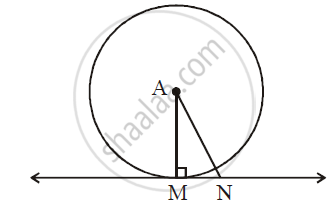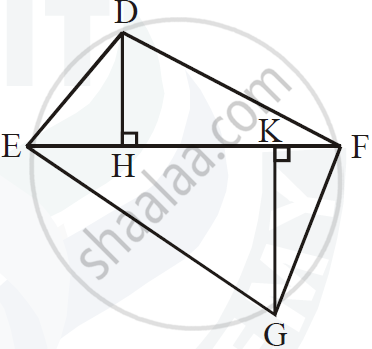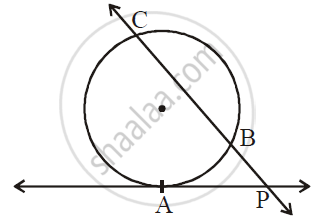# Geometry Set A 2017-2018 SSC (Marathi Semi-English) 10th Standard [इयत्ता १० वी] Question Paper Solution

Geometry [Set A]
Date & Time: 12th March 2018, 11:00 am
Duration: 2h

1 | Attempt any FIVE of the following sub-questions
1.1

triangleDEF ~ triangleMNK. If DE = 5 and MN = 6, then find the value of (A(triangleDEF))/(A(triangleMNK))

Concept: Similarity of Triangles
Chapter: [0.01] Similarity
1.2

If two circles with radii 8 cm and 3 cm respectively touch externally, then find the distance between their centres.

Concept: Touching Circles
Chapter: [0.03] Circle
1.3

Find the length of the altitude of an equilateral triangle with side 6 cm.

Concept: Construction of Triangle If the Base, Angle Opposite to It and Either Median Altitude is Given
Chapter: [0.05] Geometric Constructions
1.4

If theta = 45^@, then find tan theta.

Concept: Angles in Standard Position
Chapter: [0.06] Trigonometry
1.5

A slope of a line is 3 and y-intercept is –4. Write the equation of a line

Concept: Slope of a Line
Chapter: [0.04] Co-ordinate Geometry
1.6

Using Euler’s formula, find V if E = 30, F = 12.

Concept: Euler's Formula
Chapter: [0.07] Mensuration
2 | Attempt any FOUR of the following subquestions:
2.1

The ratio of the areas of two triangles with common base is 6:5. Height of the larger triangle of 9 cm, then find the corresponding height of the smaller triangle.

Concept: Properties of Ratios of Areas of Two Triangles
Chapter: [0.01] Similarity
2.2

In the following figure, point ‘A’ is the centre of the circle. Line MN is tangent at point M. If AN = 10 cm and MN = 5 cm, determine radius of the circle.Concept: Theorem - Converse of Tangent at Any Point to the Circle is Perpendicular to the Radius
Chapter: [0.03] Circle
2.3

Draw angle ABC of measure 80° and bisect it

Concept: Basic Geometric Constructions
Chapter: [0.05] Geometric Constructions
2.4

if cos theta = 5/13 where theta is an acute angle. Find the value of sin theta

Concept: Trigonometric Identities
Chapter: [0.06] Trigonometry
2.5

The volume of a cube is 512 cm3. Find its side.

Concept: Surface Area of a Combination of Solids
Chapter: [0.07] Mensuration
2.6

The radius and slant height of a cone are 5 cm and 20 cm respectively. Find its curved surface area. (Π = 3.14)

Concept: Surface Area of a Combination of Solids
Chapter: [0.07] Mensuration
3 | Attempt any THREE of the following subquestions
3.1

In the following figure, seg DH ⊥ seg EF and seg GK ⊥ seg EF. If DH = 18 cm, GK = 30 cm and A(triangle DEF) = 450 cm^2, then find:

1) EF

2) A(triangle GFE)

3) A(square DFGE)Concept: Similarity of Triangles
Chapter: [0.01] Similarity
3.2

In the following figure, ray PA is a tangent to the circle at A and PBC is a secant. If AP = 15, BP = 10, then find BC.Concept: Tangent - Secant Theorem
Chapter: [0.03] Circle
3.3

Draw the circle with centre C and radius 3.5 cm. Point B is at a distance 7 cm from the centre. Draw tangents to the circle from the point B.

Concept: Construction of Tangent to the Circle from the Point on the Circle
Chapter: [0.05] Geometric Constructions
3.4

Show that sqrt((1+cosA)/(1-cosA)) = cosec A + cot A

Concept: Trigonometric Identities
Chapter: [0.06] Trigonometry
3.5

Write the equation of the line passing through A(–3, 4) and B(4, 5) in the form of ax + by + c = 0

Concept: Standard Forms of Equation of a Line
Chapter: [0.04] Co-ordinate Geometry
4 | Attempt any TWO of the following subquestions
4.1

Prove that “The lengths of the two tangent segments to a circle drawn from an external point are equal.”

Concept: Number of Tangents from a Point on a Circle
Chapter: [0.03] Circle [0.03] Circle
4.2

A tree is broken by the wind. The top of that tree struck the ground at an angle of 30° and at a distance of 30. Find the height of the whole tree

Concept: Heights and Distances
Chapter: [0.06] Trigonometry
4.3

A (5, 4), B (-3, -2) and C (1, -8) are the vertices of a triangle ABC. Find the equations of the median AD and line parallel to AC passing through the point B.

Concept: General Equation of a Line
Chapter: [0.04] Co-ordinate Geometry
5
5.1

Prove that, in a right-angled triangle, the square of the hypotenuse is equal to the sum of the square of remaining two sides

Concept: Right-angled Triangles and Pythagoras Property
Chapter: [0.02] Pythagoras Theorem
5.2

ΔSHR ~ ΔSVU. In ΔSHR, SH = 4.5 cm, HR = 5.2 cm, SR = 5.8 cm and (SH)/(SV)=3/5. Construct ΔSVU.

Concept: Division of a Line Segment
Chapter: [0.04] Co-ordinate Geometry [0.05] Geometric Constructions
5.3

If ‘V’ is the volume of a cuboid of dimensions a × b × c and ‘S’ is its surface area, then prove that: 1/V = 2/S[1/a +1/b + 1/c]

Concept: Surface Area of a Combination of Solids
Chapter: [0.07] Mensuration

#### Request Question Paper

If you dont find a question paper, kindly write to us

View All Requests

#### Submit Question Paper

Help us maintain new question papers on Shaalaa.com, so we can continue to help students

only jpg, png and pdf files

## Maharashtra State Board previous year question papers 10th Standard [इयत्ता १० वी] Geometry with solutions 2017 - 2018

Maharashtra State Board 10th Standard [इयत्ता १० वी] Geometry question paper solution is key to score more marks in final exams. Students who have used our past year paper solution have significantly improved in speed and boosted their confidence to solve any question in the examination. Our Maharashtra State Board 10th Standard [इयत्ता १० वी] Geometry question paper 2018 serve as a catalyst to prepare for your Geometry board examination.
Previous year Question paper for Maharashtra State Board 10th Standard [इयत्ता १० वी] Geometry-2018 is solved by experts. Solved question papers gives you the chance to check yourself after your mock test.
By referring the question paper Solutions for Geometry, you can scale your preparation level and work on your weak areas. It will also help the candidates in developing the time-management skills. Practice makes perfect, and there is no better way to practice than to attempt previous year question paper solutions of Maharashtra State Board 10th Standard [इयत्ता १० वी].

How Maharashtra State Board 10th Standard [इयत्ता १० वी] Question Paper solutions Help Students ?
• Question paper solutions for Geometry will helps students to prepare for exam.
• Question paper with answer will boost students confidence in exam time and also give you an idea About the important questions and topics to be prepared for the board exam.
• For finding solution of question papers no need to refer so multiple sources like textbook or guides.